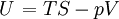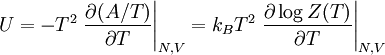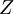2022: SklogWiki celebrates 15 years on-line

# Internal energy$\left. U \right.=TS-pV$$U=-T^2 \left. \frac{\partial (A/T)}{\partial T} \right\vert_{N,V} = k_B T^{2} \left. \frac{\partial \log Z(T)}{\partial T} \right\vert_{N,V}$
where$A$ is the Helmholtz energy function and$Z$ is the partition function.